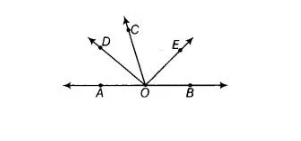# In the figure, OD is the bisector of ∠AOC,`
Question:

In the figure, OD is the bisector of ∠AOC, OE is the bisector of ∠BOC and OD ⊥ OE. Show that the points A, 0 and B are collinear.Thinking Process

For showing collinearity of A, O and B, we have to show that ∠AOB =180°.

Solution:

Given In the figure, OD ⊥ OE, OD and OE are the bisectors of ∠AOC and ∠BOC.

To show Points A, O and B are collinear i.e., AOB is a straight line.

Proof Since, OD and OE bisect angles ∠AOC and ∠BOC, respectively.

∠AOC =2 ∠DOC …(i)

and ∠COB = 2 ∠COE …(ii)

On adding Eqs. (i) and (ii), we get

∠AOC + ∠COB = 2 ∠DOC +2 ∠COE => ∠AOC +∠COB = 2(∠DOC +∠COE)

=> ∠AOC + ∠COB= 2 ∠DOE

=> ∠AOC+ ∠COB = 2 x 90° [∴ OD ⊥ OE]

=> ∠AOC + ∠COB = 180°

∴ ∠AOB = 180°

So, ∠AOC and ∠COB are forming linear pair.

Also, AOB is a straight line.

Hence, points A, O and B are collinear.